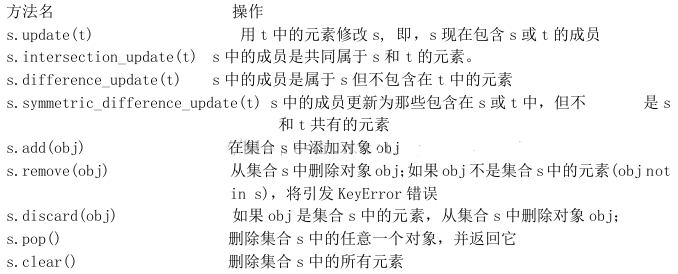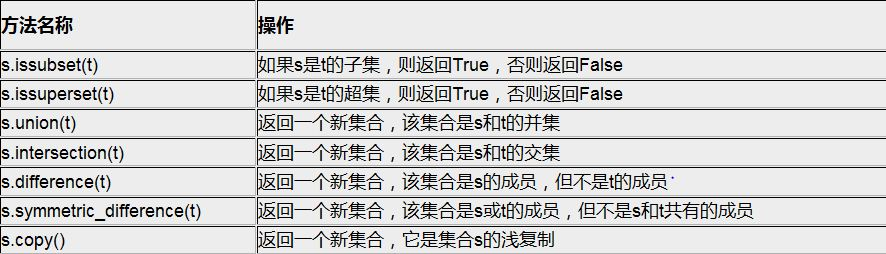### Python编程语言

Python 是一种面向对象、解释型计算机程序设计语言，由Guido van Rossum于1989年底发明，第一个公开发行版发行于1991年。Python语法简洁而清晰，具有丰富和强大的类库。它常被昵称为胶水语言，它能够把用其他语言制作的各种模块（尤其是C/C++）很轻松地联结在一起。

（1）标准类型函数

len()：把集合作为参数传递给内建函数 len()，返回集合的基数（或元素的个数）。
（2）集合类型工厂函数
set()和 frozenset()工厂函数分别用来生成可变和不可变的集合。如果不提供任何参数，默认会生成空集合。如果提供一个参数，则该参数必须是可迭代的，即一个序列或迭代器或支持迭代的一个对象，例如一个文件或一个字典。
（3）方法(所有的集合方法)
s.issubset(t)                  如果s是t的子集,则返回True,否则返回False
s.issuperset(t)               如果t是s的超集,则返回True,否则返回False
s.union(t)                       返回一个新集合,该集合是s和t的并集
s.intersection(t)            返回一个新集合,该集合是s和t的交集
s.difference(t)               返回一个新集合,该集合是 s 的成员,但不是 t 的成员
s.symmetric_difference(t)     返回一个新集合,该集合是s或t的成员,但不是s和t共有的成员
s.copy()                         返回一个新集合,它是集合s的浅复制
内建方法copy() 没有等价的操作符。和同名的字典方法一样，copy()方法比用像set()、frozenset()或dict()这样的工厂方法复制对象的副本要快。
（4）方法(仅适用于可变集合)```>>> s = set('cheeseshop')
>>> t = set('bookshop')
>>> s
set(['c', 'e', 'h', 'o', 'p', 's'])
>>> t
set(['b', 'h', 'k', 'o', 'p', 's'])
>>> s.issubset(t)
False
>>> s.issuperset(t)
False
>>> s.union(t)
set(['c', 'b', 'e', 'h', 'k', 'o', 'p', 's'])
>>> s.intersection(t)
set(['h', 's', 'o', 'p'])
>>> s.difference(t)
set(['c', 'e'])
>>> s.symmetric_difference(t)
set(['b', 'e', 'k', 'c'])
>>> s.copy()
set(['p', 'c', 'e', 'h', 's', 'o'])

```

1、s.update(t)——用t中的元素修改s，即s现在包含s或t的成员。

```>>> s.update(t)
>>> s
set(['c', 'b', 'e', 'h', 'k', 'o', 'p', 's'])

```

2、s.intersection_update(t)——s中的成员是共同属于s和t中的元素。

```>>> s = set('cheeseshop')
>>> t = set('bookshop')
>>> s.intersection_update(t)
>>> s
set(['h', 's', 'o', 'p'])

```

3、s.difference_update(t)——s中的成员是属于s但不包含在t中的元素。

```>>> s = set('cheeseshop')
>>> t = set('bookshop')
>>> s.difference_update(t)
>>> s
set(['c', 'e'])

```

4、s.symmetric_difference_update(t)——s中的成员更新为那些包含在s或t中，但不是s和t共有的元素。

```>>> s = set('cheeseshop')
>>> t = set('bookshop')
>>> s.symmetric_difference_update(t)
>>> s
set(['c', 'b', 'e', 'k'])

```

```>>> s.add('o')
>>> s
set(['c', 'b', 'e', 'k', 'o'])

```

6、s.remove(obj)——从集合s中删除对象obj，如果obj不是集合s中的元素（obj not in s），将引发KeyError。

```<p>>>> s.remove('b')
>>> s
set(['c', 'e', 'k', 'o'])
>>> s.remove('a')</p><p>Traceback (most recent call last):
File "<pyshell#53>", line 1, in <module>
s.remove('a')
KeyError: 'a'
</p>

```

```>>> s.discard('a')
>>> s
set(['c', 'e', 'k', 'o'])
>>> s
set(['c', 'k', 'o'])

```

8、s.pop()——删除集合是中的任意一个对象，并返回它。

```>>> s.pop()
'c'
>>> s
set(['k', 'o'])

```

9、s.clear()——删除集合s中的所有元素。

```>>> s.clear()
>>> s
set([])

```

Python中集合的内建函数和内建方法学习教程
Python中3种内建数据结构：列表、元组和字典应用讲解
Python中lambda的用法和def的区别

Python中type的构造函数参数含义说明
python 回调函数和回调方法的实现分析

Python的shutil模块中文件的复制操作函数详解
python中redis的安装和使用
Python中装饰器兼容加括号和不加括号的写法详解
Python中使用PyHook监听鼠标和键盘事件实例代码
python中enumerate的用法讲解